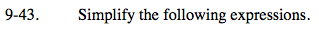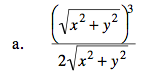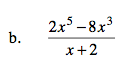### Home > AC > Chapter 16 > Lesson 16.9.1.3 > Problem9-43

9-43.$\textit{u} = \sqrt{\textit{x}^2 + \textit{y}^2}$

$\text{Now: } \frac{u^3}{2u} = \frac{u^2}{2}$

Substitute the value of 'u' back in.1. Factor out a 2x3 in the numerator.
2. Factor the remaining binomial in the numerator.
3. Simplify.